# Problem 53725. Easy Sequences 56: Counting "Ugly" Numbers

A positive integer x is called a regular number, if and only if there exist a non-negative integer k, such that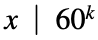. For some reason, such a number is also refered to as an ugly number. Below are the first few regular numbers: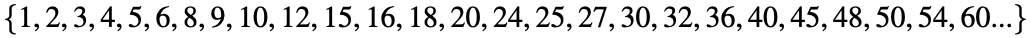It turns out that regular numbers are not so regular after all. In fact, regular numbers are quite rare. There are only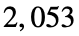regular numbers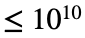and just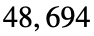regular numbers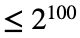.
Given an integer n, and exponent e, we are tasked to write a function that counts the number of regular numbers less than or equal to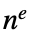.

### Solution Stats

60.0% Correct | 40.0% Incorrect
Last Solution submitted on Jul 01, 2023

### Community Treasure Hunt

Find the treasures in MATLAB Central and discover how the community can help you!

Start Hunting!